## LODs vs Table Calcs: How to compare the profits of all the Sub-categories to that of the selected one?

by Manuela Marolla

##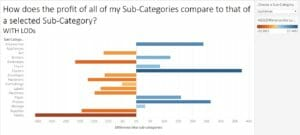In this example, we are going to look at two different ways to get the same result in Tableau.

Follow along using Tableau’s Superstore dataset.

Look at the screenshot above. It’s the final result that we want to achieve.

We want to compare the profit of each Sub-Category to the one of the Sub-Category selected by the Parameter on the right.

As you can see, the value for Appliances is 0 in the view. This is because the profit for each Sub-Category is compared to that one.

## Steps:

1. Let’s right-click on the Sub-Category dimension and create a parameter called Choose a Sub-Category.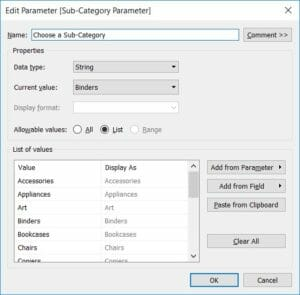Then click on Show Parameter Control.

Now we want to look at the Accessories’ profit (the first item under Sub-Category) and compare it to the Envelopes’ profit, which we have selected in the Parameter, and we want to repeat this process for all the other items.

Therefore, we first need to calculate the Envelopes’ profit, which we don’t know.

The LOD below allows you to bring back the total profit for the Sub-Category selected through the Parameter Choose a Sub-Category.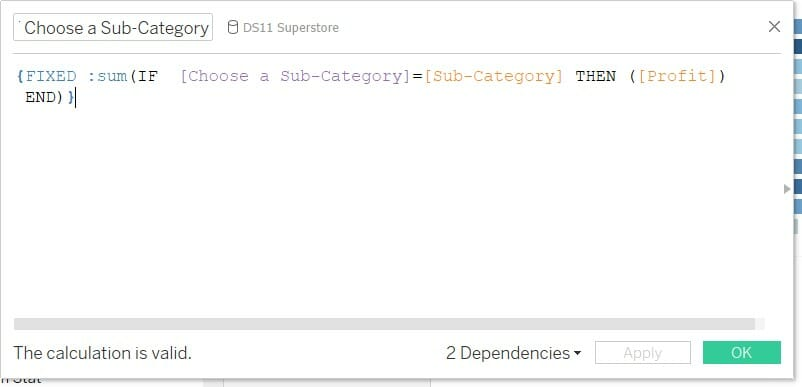Notice how the LOD isn’t fixed on any dimension.

In this case,  {FIXED or { not followed by anything would mean the same thing.

Now that we have assigned a value for the selected Sub-Category, we can calculate the difference between the SUM(Profit) of each Sub-Category and the one of the Sub-Category we have selected via the parameter.

To do that, we calculate the difference between the SUM(Profit) and the LOD calculation. I have called this calculated field Difference between sub-categories.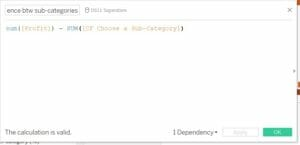Drag Sub-Category onto Rows and this calculated field both onto Columns and the Color Shelf and you will be able to see how the profit of all the Sub-Categories compare to that of Appliances.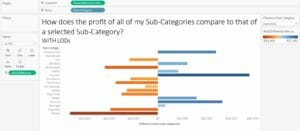## Are LODs the only way to solve it? Actually, no. Let’s try using Table Calcs

Since we have all the fields in the view, we can replace LODs with Table Calcs.

Again, let’s drag Sub-Category onto Rows and SUM(Profit) onto both Columns and the Color shelf.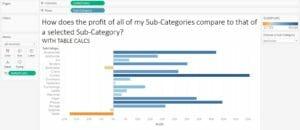Now we want to add a Table Calculation to calculate the difference.

Let’s right-click on SUM(Profit), select Quick Table Calculation and then Difference.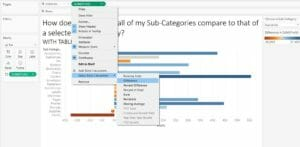However, we still need to configure the Table Calculation correctly.

Click on Edit Table Calculation. Compute using Specific Dimensions and check Sub-Category.

This time, we need to compute the difference relative to the parameter. Select Choose the Sub-Category parameter from the drop-down.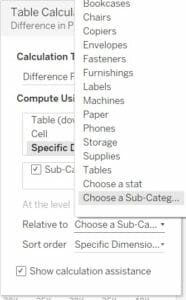You have now have obtained the same chart as above, but without using LODs.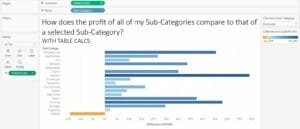Manuela Marolla